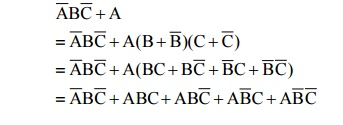Home | | Digital Electronics | Standard SOP Form & Minterms

# Standard SOP Form & Minterms

SOP expressions containing all Variables in the Domain in each term are in Standard Form.

STANDARD SOP FORM & MINTERMS:

â€˘               SOP expressions containing all Variables in the Domain in each term are in Standard Form.

â€˘               Standard product terms are also called Minterms.

â€˘               Any non-standard SOP expression may be converted to Standard form by applying Boolean Algebra Rule 6 to it.

Example:Example: Determine Standard SOP expressionIntroduce all possible combinations of the missing variables ANDâ€™ed with the original term

## CHARACTERISTICS OF A MINTERM:

â€˘               Minterm is a standard product term in which all variables appear exactly once (complemented or uncomplemented)

â€˘               Represents exactly one combination of the binary variables in a truth table for which the function produces a â€ś1â€ť output. That is the binary representation or value.

â€˘               Has value of 1 for that combination and 0 for all others

â€˘               For n variables, there are 2n distinct minterms

Example:Express the Boolean function F=A+Bâ€˛C in sum of min terms.

Given

F=A+Bâ€˛C

=A(B+ Bâ€˛)(C+Câ€˛)+ Bâ€˛C(A+Aâ€˛)

=(AB+A Bâ€˛)(C+Câ€˛)+Bâ€˛C(A+Aâ€˛)

=ABC+ABCâ€˛+ABâ€˛C+ABâ€˛Câ€˛+ABâ€˛C+Aâ€˛Bâ€˛C

= ABC+ABCâ€˛+ABâ€˛C+ABâ€˛C+Aâ€˛Bâ€˛C

F=m1+m4+m5+m6+m7

Study Material, Lecturing Notes, Assignment, Reference, Wiki description explanation, brief detail
Digital Electronics : Minimization Techniques and Logic Gates : Standard SOP Form & Minterms |